Courses

# Fluid Mechanics, Strength Of Materials - 2

## 20 Questions MCQ Test GATE Mechanical (ME) 2022 Mock Test Series | Fluid Mechanics, Strength Of Materials - 2

Description
This mock test of Fluid Mechanics, Strength Of Materials - 2 for GATE helps you for every GATE entrance exam. This contains 20 Multiple Choice Questions for GATE Fluid Mechanics, Strength Of Materials - 2 (mcq) to study with solutions a complete question bank. The solved questions answers in this Fluid Mechanics, Strength Of Materials - 2 quiz give you a good mix of easy questions and tough questions. GATE students definitely take this Fluid Mechanics, Strength Of Materials - 2 exercise for a better result in the exam. You can find other Fluid Mechanics, Strength Of Materials - 2 extra questions, long questions & short questions for GATE on EduRev as well by searching above.
QUESTION: 1

Solution:
QUESTION: 2

Solution:
QUESTION: 3

### Which one of the following velocity fields represents a possible fluid flow?

Solution:
QUESTION: 4

Two long columns C1 and C2 are made of the same material. Column C1 has both the ends hinged while column C2 has one end hinged and the other end fixed. What is the ratio of the critical load for C1 to that for C2 according to Euler’s formula?

Solution:
QUESTION: 5

From a cicular plate of diameter D,whose center is C,  another cicular plate of diameter AC is cut ,removed and kept over CB,The thickness of the plate is uniform. The shift of the centroid due to this will be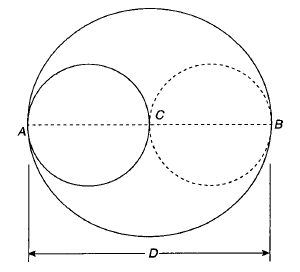Solution:
QUESTION: 6

A cantilever is subjected to a uniformly distributed load W over its whole length L and a concentrated upward force W at its free end. The deflection of the free end is

Solution:
QUESTION: 7

The deformation of the bar under its own weight as compared to that when subjected to a direct axial load equal to its own weight will be

Solution:
QUESTION: 8

A thin cylindrical shell of internal diameter D with t is subjected to internal pressure p. The change in diameter is

Solution: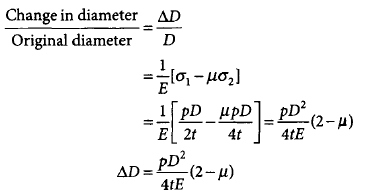QUESTION: 9

A material having modulus of elasticity 200 GN/m2 and Poissons ratio 0.25 will have modulus of rigidity

Solution: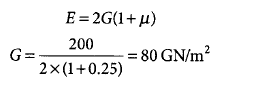*Answer can only contain numeric values
QUESTION: 10

A simply supported beam AB is loaded as shown in the figure below.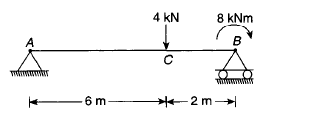What is the shear force (SF) in kN in the portion AC of the beam?

Solution:
*Answer can only contain numeric values
QUESTION: 11

A shaft is initially subjected to bending moment and then to torsion. In that case, if the magnitude of bending moment is found to be the same as that of torque, then the ratio of maximum bending stress to shear stress would be

Solution:
QUESTION: 12

A steel rod, 100 mm long, is held between two rigid supports. It is heated to 20°C. If the coefficient of thermal expansion of the material of the rod is 15 x 10-6/°C and the modulus of elasticity is 200 x 103 MN/m2, what is the stress in the rod?

Solution: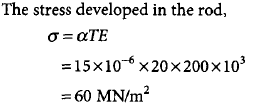QUESTION: 13

A mild steel bar of square cross-section 40 mm x 40 mm is 400 mm long. It is subjected to a longitudinal tensile stress of 440 N/mm2 and a lateral compressive stress of 200 N/mm2 in perpendicular directions. E = 2 x 10-5 N/ mm2, μ = 0.3. What is the approximate elongation of the bar in the longitudinal direction?

Solution: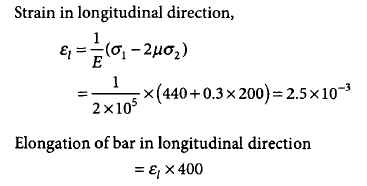= 2.5 x 10-3 x 400  = 1mm

QUESTION: 14

At a certain point in a structural member, there are perpendicular stresses 80 N/mm2 and 20 N/mm2, both tensile. What is the equivalent stress in simple tension, according to the maximum principal strain theory? (Poisson’s ratio = 0.25)

Solution: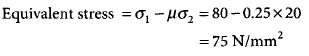QUESTION: 15

The free end of a cantilever beam is supported by the free end of another cantilever beam using a roller as shown in the figure given below. What is the deflection at the roller support B?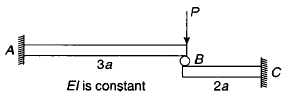Solution: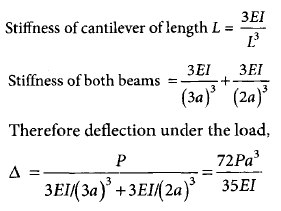QUESTION: 16

Mohar's Circle given below corresponds to which one of the following stress conditions?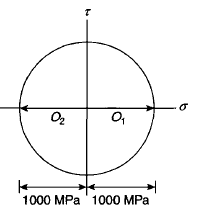Solution:
QUESTION: 17

A 2-m long rod at a temperature of 10°C is allowed to expand with temperature rising to 80°C. (E = 100,000 MN/m2 and X= 0.000012°C)

QThe expension of the rod due to the rise in temperature will be

Solution:
QUESTION: 18

A 2-m long rod at a temperature of 10°C is allowed to expand with temperature rising to 80°C. (E = 100,000 MN/m2 and X= 0.000012°C)

Q. The stress in the rod, if expansion is not allowed, will be

Solution:
QUESTION: 19

Match list I with list II and select the correct answer using the codes given below: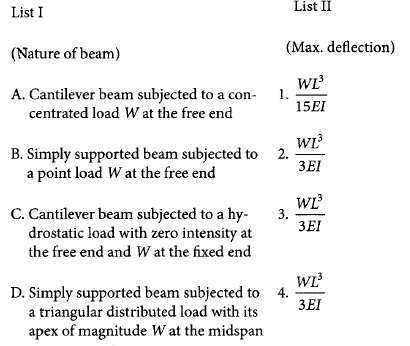Codes: ABCD

Solution:
QUESTION: 20

Match list I with list II and select the correct answer using the codes given below:
List I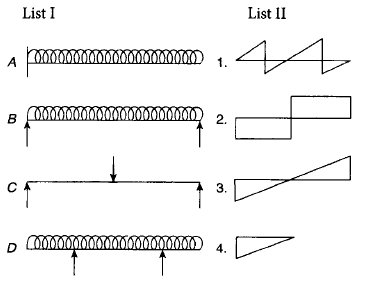Codes: ABCD

Solution: